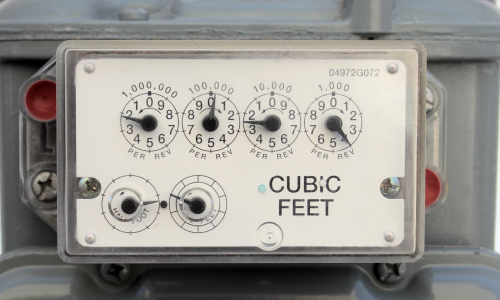# Gas kWh Conversion Tool

## Need to convert your gas units in cubic feet or metres into kilowatt-hours?

Use this simple tool to perform a conversion based on your latest gas bills or meter readings.

We have pre-filled the form with default values for Correction Factor and Calorific Value, so simply enter the number of units in hundreds of cubic feet (ft3(x100)) or cubic metres (m3) and press ‘Calculate’.

## Gas kWh Calculator

Enter the number of units as shown on your bill, or as calculated from a recent meter reading.
Note that imperial meters usually count hundreds of cubic feet, so that is the format this calculator requires.

Your estimated number of kilowatt-hours used will be displayed below:

kWh

Subtract your latest meter reading from the one on your last bill to get a figure for gas used, but not yet paid for.

Example of a metric meter with digital display. This reading would be 12,757m3:(Digital display on a metric meter. Read only the black digits and ignore and numbers in red or after a decimal point)

Example of an imperial meter with dial display. This reading would be 2,024,000ft3 (this meter records thousands ft3):(Imperial meter with dials increasing in value from right to left. Ignore any red dials, and use the lower figure when the dial is between two digits (except when it is between 0 and 9, in which case use 9))

Disclaimer: This page is provided as a tool, and it is up to the user to ensure its output is correct. We advise you to check any calculations by hand once completed.

Metric formula: (No. of cubic metres x Correction Factor x Calorific Value) / 3.6

Imperial formula: (Hundreds of cubic feet x 2.83 x Correction Factor x Calorific Value) / 3.6